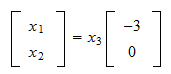StatLect

# Homogeneous system

A homogeneous system of equations is a system in which the vector of constants on the right-hand side of the equals sign is zero.

In this lecture we provide a general characterization of the set of solutions of a homogeneous system.## Definition

A homogenous system has the formwhereis amatrix of coefficients,is avector of unknowns andis thezero vector.

Example The systemwhich can be written in matrix form asis homogeneous.

## Equivalent system in row echelon form

By performing elementary row operations on a homogenous system, we obtain equivalent systems that are all homogenous. In fact, elementary row operations (multiplying an equation by a non-zero constant; adding a multiple of one equation to another equation; interchanging two equations) leave the zero vector of constants on the right-hand side of the equals sign unaffected.

As a consequence, we can transform the original system into an equivalent homogeneous systemwhere the matrixis in row echelon form (REF).

## Trivial solution

A homogeneous system always has the solutionwhich is called trivial solution.

## Basic and non-basic variables

Remember that the columns of a REF matrix are of two kinds:

• basic columns: they contain a pivot (i.e., a non-zero entry such that we find only zero entries in the quadrant starting from the pivot and extending below it and to its left);

• non-basic columns: they do not contain a pivot.

Example Consider the followingmatrix in row echelon form:The first and the third columns are basic, while the second and the fourth are non-basic.

## Partitioned system

Suppose that theREF matrixhasbasic columns.

Without loss of generality, we can assume that the firstcolumns are basic and the lastare non-basic (we can re-number the unknowns if necessary).

Partition the matrixinto two blocks:whereis thesub-matrix of basic columns andis thesub-matrix of non-basic columns.

Similarly, partition the vector of unknowns into two blocks:whereis thevector of basic variables andis thevector of non-basic variables.

Then, we can write the system of equations as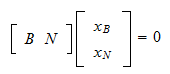or## General solution

The general solution of the homogeneous systemis the set of all possible solutions, that is, the set of allthat satisfy the system of equations.

We already know that, if the system has a solution, then we can arbitrarily choose the values of the non-basic variablesand then find, by the back-substitution algorithm, the values of the basic variablesthat solve the system. In the homogeneous case, the existence of a solution is not an issue because the vector of constants is zero (revise the lecture on the row echelon form if you are wondering why).

Therefore, there is a uniquethat solves equation (1) for any arbitrary choice of.

Sinceis full-rank and, the matrixis full-rank (see the lecture on the rank of matrix products). Therefore, we can pre-multiply equation (1) byso as to obtain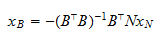DefineThen, we haveThe latter can be used to characterize the general solution of the homogeneous system: it explicitly links the values of the basic variables to those of the non-basic variables that can be set arbitrarily.

Denote bythe general solution (i.e., the set of all possible solutions). Then, we have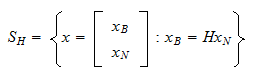The productcan be seen as a linear combination of the columns ofwhose coefficients are the non-basic variables: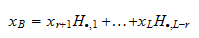Thus, each column ofis a particular solution of the system, obtained by setting its corresponding non-basic variable equal toand all the other non-basic variables equal to. By taking linear combination of these particular solutions, we obtain the general solution.

Clearly, the general solution embeds also the trivial one, which is obtained by setting all the non-basic variables to zero.

Example Consider the homogeneous systemwhere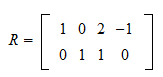andThen, we can defineThe system can be written asbut sinceis the identity matrix, we haveThus, the general solution of the system is the set of all vectorsthat satisfy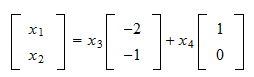## Solved exercises

Below you can find some exercises with explained solutions.

### Exercise 1

Define thematrixFind the general solution of the systemwhereis avector of unknowns.

Solution

The matrixis not in row echelon form, but we can subtract three times the first row from the third one in order to obtain an equivalent matrix in row echelon form:Thus, we can discuss the solutions of the equivalent systemSince both of the two columns ofare basic, there are no unknowns to choose arbitrarily. As a consequence, the only solution of the system is the trivial one ().

### Exercise 2

Define thematrixFind the general solution of the systemwhereis avector of unknowns.

Solution

For convenience, we are going to transforminto a reduced row echelon form matrix. We divide the second row by; then, we subtract two times the second row from the first one. The result is an equivalent matrix in reduced row echelon form:We can now discuss the solutions of the equivalent systemThe system can be written as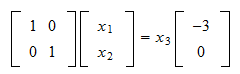Thus, the general solution of the system is the set of all vectorsthat satisfy